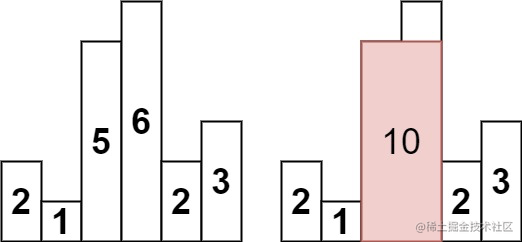# LeetCode - 84. 柱状图中最大的矩形```java class Solution { public int largestRectangleArea(int[] heights) { int n = heights.length; if (n == 0) { return 0; } if (n == 1) { return heights; } int[] newHeights = new int[n + 2]; System.arraycopy(heights, 0, newHeights, 1, n); heights = newHeights; n += 2; int max = 0; Deque<Integer> stack = new LinkedList<>(); stack.push(0); for (int i = 1; i < n; i++) { while (heights[stack.peek()] > heights[i]) { int h = heights[stack.pop()]; while (!stack.isEmpty() && heights[stack.peek()] == h) { stack.pop(); } int w = i - stack.peek() - 1; max = Math.max(max, w * h); } stack.push(i); } return max; } }```